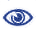# The Prague Stringology Conference 2009

Finding all covers of an indeterminate string in O(n) time on average

 Abstract: We study the problem of finding all the covers of an indeterminate string. An indeterminate string is a sequence T = TT...T[n], where T[i] ⊆ Σ for each i, and Σ is a given alphabet of fixed size. Here we describe an algorithm for finding all the covers of a string x. The algorithm is applicable for both regular and indeterminate strings. Our algorithm starts with the border array and uses pattern matching technique of the Aho-Corasick Automaton to compute all the covers of x from the border array. On average the algorithm requires O(n) time to find out all the covers, where n is the length of x. Finally, we extend our algorithm to compute the cover array of x in O(n2) time and O(n) space complexity.

 Download paper:PostScript PDF BibTeX reference
 Download presentation: